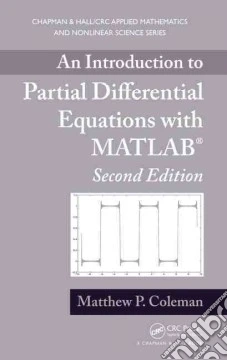ricerca
avanzata

# An Introduction to Partial Differential Equations With MATLAB

## Un libro in lingua di Coleman Matthew P. edito da Chapman & Hall, 2013

• € 73,00
• Il prezzo è variabile in funzione del cambio della valuta d’origine"Preface Many problems in the physical world can be modeled by partial differential equations, from applications as diverse as the flow of heat, the vibration of a ball, the propagation of sound waves, the diffusion of ink in a glass of water, electric and magnetic fields, the spread of algae along the ocean's surface, the fluctuation in the price of a stock option, and the quantum mechanical behavior of a hydrogen atom. However, as with any area of applied mathematics, the field of PDEs is interesting not only because of its applications, but because it has taken on a mathematical life of its own. The author has written this book with both ideas in mind, in the hope that the student will appreciate the usefulness of the subject and, at the same time, get a glimpse into the beauty of some of the underlying mathematics. This text is suitable for a two-semester introduction to partial differential equations and Fourier series for students who have had basic courses in multivariable calculus (through Stokes's and the Divergence Theorems) and ordinary differential equations. Over the years, the author has taught much of the material to undergraduate mathematics, physics and engineering students at Penn State and Fairfield Universities, as well as to engineering graduate students at Penn State and mathematics and engineering graduate students at Fairfield. It is assumed that the student has not had a course in real analysis. Thus, we treat pointwise convergence of Fourier series and do not talk about mean-square convergence until Chapter 8 (and, there, in terms of the Riemann, and not the Lebesgue, integral). Further, we feel that it is not appropriate to introduce so subtle an idea as uniform convergence in this setting, so we discuss it only in the Appendices"--